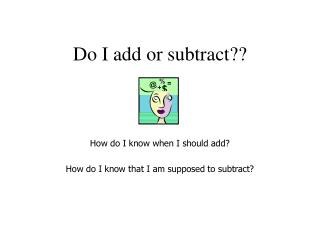Download Presentation# Do I add or subtract??

Download Presentation## Do I add or subtract??

- - - - - - - - - - - - - - - - - - - - - - - - - - - E N D - - - - - - - - - - - - - - - - - - - - - - - - - - -
##### Presentation Transcript

1. Do I add or subtract?? How do I know when I should add? How do I know that I am supposed to subtract?

2. 3 + 4 = 7 7 + 2 = 9 4 + 2 = 6 5 + 7 = 12 7 - 2 = 5 9 - 2 = 7 3 - 1 = 2 6 - 3 = 3 Look at these problems. What is the pattern?

3. The number starts small and gets larger. The largest number is at the end. Clue words: in all more together The number starts big and gets smaller. The largest number is at the beginning. Clue words: away left Adding Subtracting

4. Try these! • 7 ____ 2 = 9 • Start at 7. • It gets larger! (7 went to 9) • The largest number (9) is at the end. • We ADD!!!

5. Can you do this one? • 8 ______ 2 = 6 • Start at 8. • It gets smaller! (8 went to 6) • The largest number is at the beginning. • We SUBTRACT!!!

6. More challenges… • 5 _____ 5 = 10 • 5 _____ 2 = 3 • 6 _____ 2 = 8 • 4 _____ 1 = 3

7. Adding The number gets larger. The largest number is at the end. Clue words: in all together more Subtracting The number gets smaller. The largest number is in the beginning. Clue words: away left Let’s review…

8. Congratulations!! • You are an expert! • Use these rules to help you decide whether to ADD or subtract. • Don’t get tricked by the math monster!